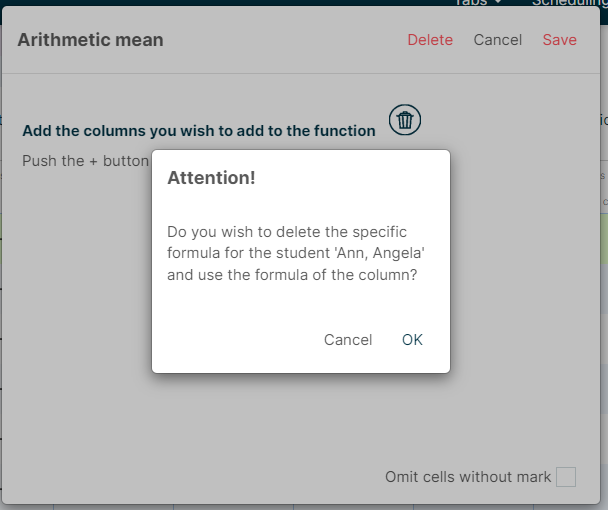1. Help center - Additio App
3. Step-by-step guide to a great setup

# Master basic calculations

### Averages and calculations:

When you create a new column, you can click on Type of evaluation > Calculations: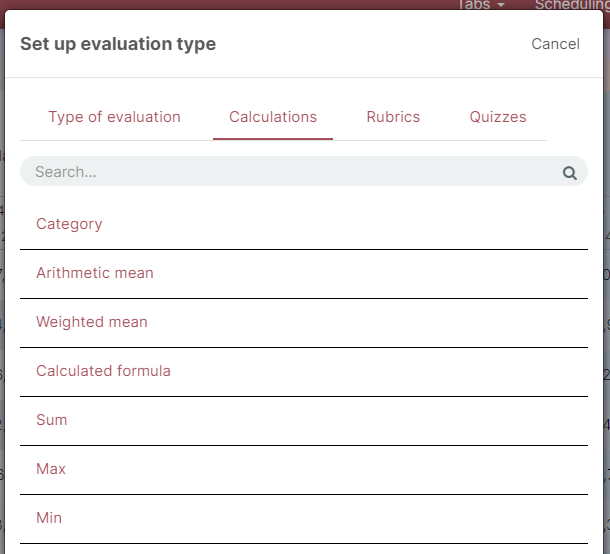You can choose the calculation you need, whether it's an arithmetic mean, weighted mean, calculated formula, sum, category, etc.

You can learn how to do calculations here:

- Weighted mean: to calculate the average of the columns you want to include and assign a weight in percentage.

Once you've selected this option, you can click on the + button, choose a column and establish its weight in percentage.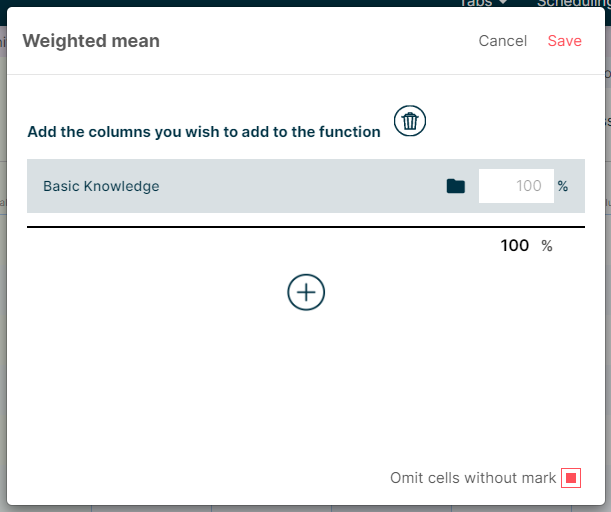You can repeat the same process to add as many columns as you may need, by clicking the + button. Do not forget the sum of the percentages must be a total of 100%.

Once you have all the values introduced click on Save.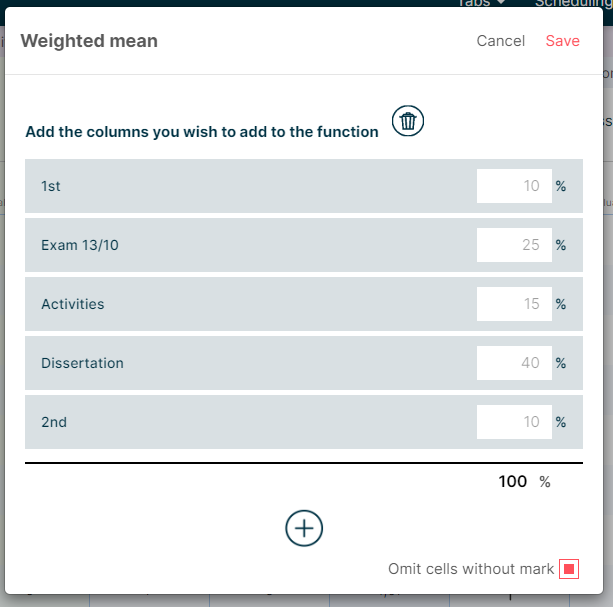NOTE: In the pop-up window where you set the weighted mean, there is a verification cell Omit cells without mark. When you check this box, the average will include only the columns with data.

Arithmetic mean: to calculate the average of all the column's selected items. A sum of all items is divided by the number of columns included.

The process is similar to Weighted mean, you need to click on the + button to select the columns you want to include in the function.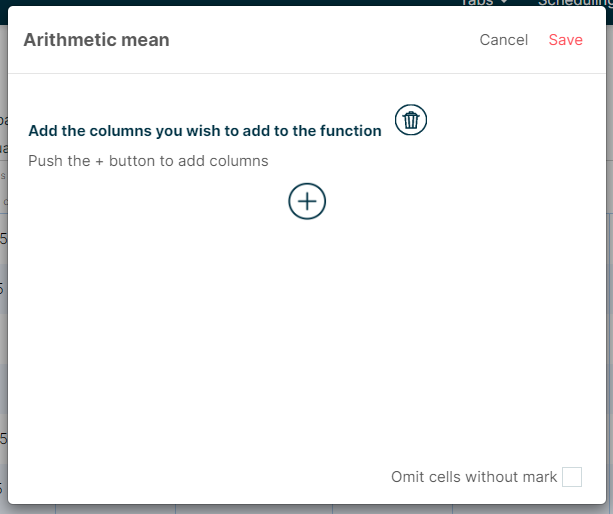NOTE: In the pop-up window where you set the arithmetic mean, there is a verification cell Omit cells without mark. When you check this box, the average will include only the columns with data.

-  Sum: as indicated in the name, it sums the values of the selected columns.

When you click the + button, you'll be able to select the desired columns in order to sum the related values.

-  Max: to establish the highest value of the selected columns.

When you click the + button, you'll be able to select the desired columns in order to find the highest value.

-  Min: to establish the lowest value of the selected columns.

When you click the + button, you'll be able to select the desired columns in order to find the lowest value.

IMPORTANT

You can copy the formula by clicking on the name of the column with the right button and Copy. Then, press the right button on the column you want to paste the formula to and Paste as link.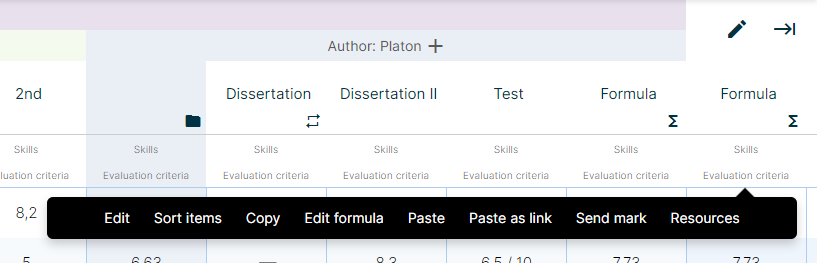### Create a calculated formula:

The type of evaluation Calculated formula enables us to do calculations of the columns desired.

As explained in the tutorial Create a column, we can set the configuration of the columns because of types of evaluation window, specifically with calculated formula we can define calculations with the Formula generator.

You can start by creating a new column and assigning the type of evaluation Calculated formula, just go to Calculations and you'll find it there:Once the formula is set, a pop-up window will emerge, and you'll be able to define the formula easily.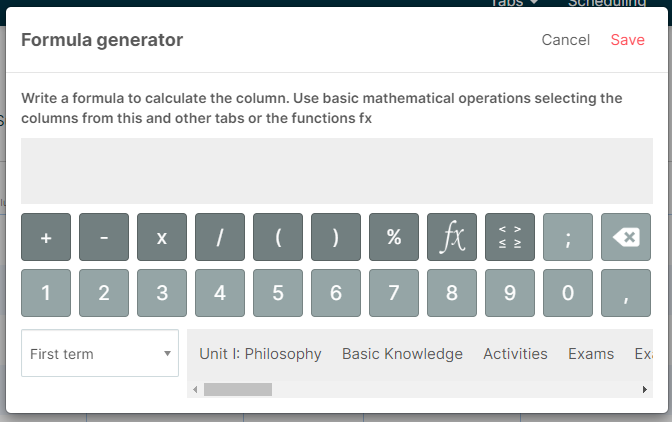To introduce the columns in the formula manually you can click on the drop-down box on the lower left side and choose a tab from the list that includes the columns you want to use. Once set, press Save to implement the changes.

### Sum of icons' values:

Let's consider we've been collecting our students attitude on a daily basis with Type of evaluation > Icon picker: faces and we want a summary of the icons' values to apply it to the final grade.

NOTE: In these cases we suggest to activate Quick columns option.

NOTE: It is important to establish the proper value for each icon from the beginning. You can set this up on Type of evaluation.

To get a count, create a new column Sum type and press the + button to add the corresponding columns of the Attitude tab.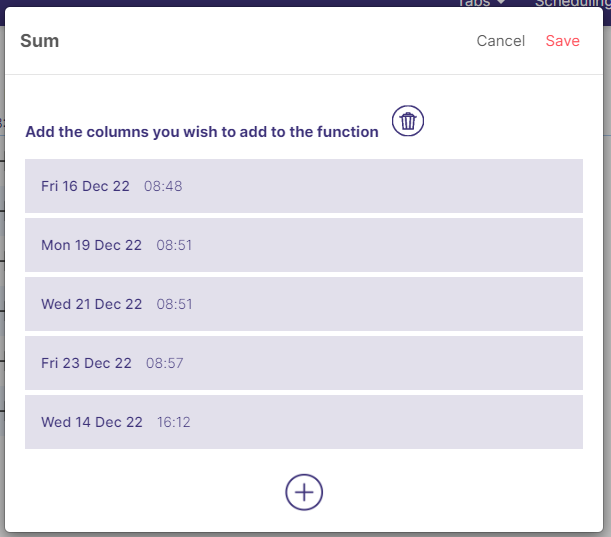Once you save the changes a new column with the sum of the icons' values will be displayed.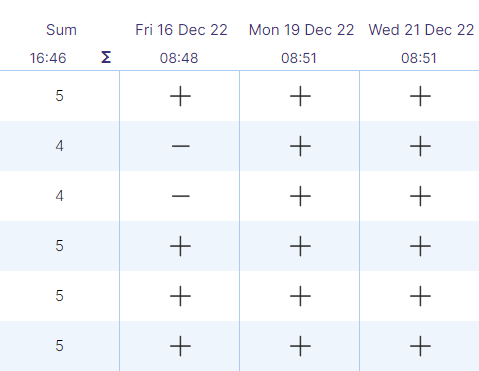### Overwrite mark:

The option Overwrite mark is displayed when you press the right button on the concerning cell, or press and hold on the cell on the app.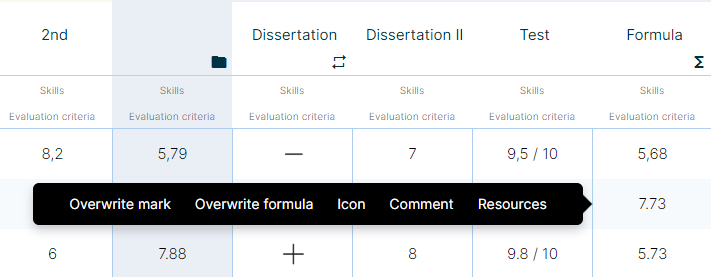### Overwrite formula:

Sometimes some students require a different assessment than others, this is why you can overwrite a formula in order to calculate and adapt easily the assessment to a particular student.

To do so, you just need to press the right button on the concerning cell, or press and hold on the cell on the app.Once you've clicked on Overwrite formula, you'll be able to modify a formula for an specific student. Click on Save to save the changes.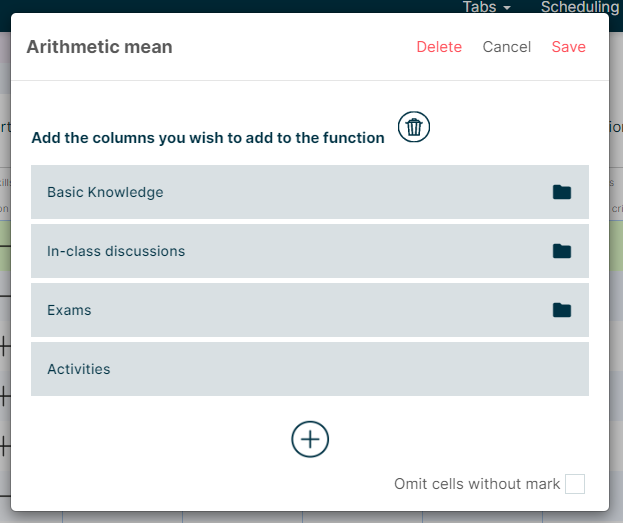Press Delete to remove the specific formula for a particular student and retrieve the original formula.